https://doi.org/10.5194/amt-16-235-2023
https://doi.org/10.5194/amt-16-235-2023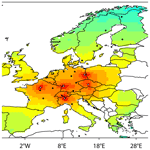# A flexible algorithm for network design based on information theory

Rona L. Thompson and Ignacio Pisso
Abstract

A novel method for atmospheric network design is presented, which is based on information theory. The method does not require calculation of the posterior uncertainty (or uncertainty reduction) and is therefore computationally more efficient than methods that require this. The algorithm is demonstrated in two examples: the first looks at designing a network for monitoring CH4 sources using observations of the stable carbon isotope ratio in CH4 (δ13C), and the second looks at designing a network for monitoring fossil fuel emissions of CO2 using observations of the radiocarbon isotope ratio in CO2 (Δ14CO2).

Share
Dates
1 Introduction

The optimal design of any observing network is an important problem in order to maximize the information obtained with minimal cost. In atmospheric sciences, observing networks include those for weather prediction, as well as for air quality and the monitoring of greenhouse gases (GHGs). For air quality and GHGs, one essential purpose of the observation network is to learn about the underlying sources and, where relevant, the sinks. This application is based on inverse methodology in which knowledge about some unknown variables, in this case the sources (and sinks), can be determined by indirect observations, that is, the atmospheric concentrations or mixing ratios, if there is a model or function that relates the unknown variables to the observations. Inverse methodology provides a means to relate the observations to the unknown variables and provides an optimal estimate of these (Tarantola, 2005).

In atmospheric sciences, the methodology is most often derived from Bayes' theorem, which describes the conditional probability of the state variables, x, given the observations, y.

$\begin{array}{}\text{(1)}& P\left(x|y\right)=\frac{P\left(y|x\right)P\left(x\right)}{P\left(y\right)}\end{array}$

Assuming a Gaussian probability density function (PDF), the following cost function can be derived (Rodgers, 2000).

$\begin{array}{}\text{(2)}& \begin{array}{rl}J\left(\mathbit{x}\right)& =\frac{\mathrm{1}}{\mathrm{2}}{\left(\mathbit{x}-{\mathbit{x}}_{\mathrm{b}}\right)}^{\mathrm{T}}{\mathbf{B}}^{-\mathrm{1}}\left(\mathbit{x}-{\mathbit{x}}_{\mathrm{b}}\right)\\ & +\frac{\mathrm{1}}{\mathrm{2}}{\left(\mathbit{H}\left(\mathbit{x}\right)-\mathbit{y}\right)}^{\mathrm{T}}{\mathbf{R}}^{-\mathrm{1}}\left(\mathbit{H}\left(\mathbit{x}\right)-\mathbit{y}\right)\end{array}\end{array}$

The x for which J(x) is the minimum is the state vector that minimizes the sum of two distances: one in the observation space, between the modelled, H(x), and observed, y, variables, and the other in the state space, between x and a prior estimate of state variables, xb. These two distances are weighted by the matrices R and B, which are, respectively, the observation error covariance and prior error covariance. Expressions for the centre and variance of the posterior PDF of x are given by e.g. Tarantola (2005).

The choice of the locations for the observations has important consequences for how well the state variables can be constrained. Increasing the number of observations will decrease the dependence of the solution on xb, but where those observations are made is also a critical consideration and depends on how they relate to the state variables, as described by the transport operator, H(x). Here only the linear transport case is considered in which this operator can be defined as the matrix H.

In practical applications of network design, there is usually a predefined budget that would allow the establishment of a given number of sites, either to create a new network or to add to an existing one. The possible locations of sites are usually a predefined set, since these need to fulfil certain criteria, e.g. access to the electrical grid, internet connection, road access, an existing building on site to house instruments, and the agreement of the property owner, and may include having an existing tower if measurements are to be made above the surface layer. Thus, the question is often the following: which potential sites should be chosen to provide the most information about the sources and sinks?

The founding work on network design was actually in the field of seismology (Hardt and Scherbaum, 1994), but there are already a number of examples of network design in the framework of atmospheric monitoring in the scientific literature. An early example is the optimization of a global network for CO2 observations to improve knowledge of the terrestrial CO2 fluxes (Gloor et al., 2000; Patra and Maksyutov, 2002; Rayner et al., 1996). These studies dealt only with small dimensional problems, i.e. with few state variables, relatively low-frequency observations, and thus small B and H matrices, and the criteria by which the network was chosen involved minimizing the posterior uncertainty. Gloor et al. (2000)​​​​​​​ solved the problem using a Monte Carlo method (specifically simulated annealing), but they found this method took considerable time to converge and up to 5 × 105 iterations were needed. Patra and Makysutov (2002) used a less computationally demanding approach, the incremental optimization method, which is based on the “divide and conquer” algorithm principle. In this method, the problem to solve is broken down into steps, i.e. sequentially choosing the best site from the set of potential sites and correspondingly depleting this set by one with each step. In the incremental optimization approach only $\sum _{i=\mathrm{1}}^{k}\left(p-i+\mathrm{1}\right)$ calculations are needed, where k is the number of sites to select and p the number of potential sites to choose from. The incremental optimization approach, however, may lead to a different selection of sites compared to testing all possible combinations of sites, which would involve $p\mathrm{!}/\left(k\mathrm{!}\left(p-k\right)\mathrm{!}\right)$ calculations, but this in many cases may be a prohibitively large number.

More recently, the problem of network design has been addressed in the context of regional networks for GHG observations (Lucas et al., 2015; Nickless et al., 2015). Again, in both these studies the metric for selecting the network was the posterior uncertainty, either by using the trace of the posterior error covariance matrix, which is equivalent to minimizing the mean square uncertainty for all grid cells (Lucas et al., 2015), or by minimizing the sum of the posterior error covariance matrix (or submatrix for a particular region), which also accounts for the covariance of uncertainty between grid cells (Nickless et al., 2015). These studies both used Monte Carlo approaches (specifically, genetic algorithms) to find the network minimizing the selected metric.

However, for large problems any metric involving the posterior uncertainty becomes a bottleneck, if not unworkable, since the calculation of the posterior error covariance matrix, A, requires inverting the matrix ${\mathbf{H}}^{\mathrm{T}}{\mathbf{R}}^{-\mathrm{1}}\mathbf{H}+{\mathbf{B}}^{-\mathrm{1}}$ which has dimensions of n×n where n is the number of state variables. For this reason, methods were proposed based on criteria considering how well a network resolves the atmospheric variability or “signal” or, in other words, how well they sample regions of significant atmospheric heterogeneity (Shiga et al., 2013). In this approach, the atmospheric signal (e.g. mixing ratio) is modelled using an atmospheric transport model and a prior flux estimate, and sites are sequentially added to the network so that the distance of any grid cell from an observation site is within some predetermined correlation scale length. For this method, the number of calculation steps is equal to the sites to be selected (Shiga et al., 2013). Although computationally very efficient, this method does not consider the information gained about the state variables but only the optimal sampling of atmospheric variability.

An alternative method, but also based on the consideration of atmospheric variability, is to consider how “similar” the atmospheric signal is between potential sites in a network and to reduce the number of sites, leaving only those with significantly different signals (Risch et al., 2014). Risch et al. (2014)​​​​​​​ applied a clustering method to cluster sites with similar signals (i.e. strongly correlated sites), and individual sites were removed from each cluster based on the premise that they did not contribute any significant new information, whereas sites in clusters of one member were all retained. However, as in the method of Shiga et al. (2013), this approach does not consider the information gained about the state variables and how atmospheric transport alone may influence the variability at each site.

Here a method for network design is proposed based on information theory. This method requires precomputed transport operators for each potential site, so-called site “footprints” or “source–receptor relationships” (SRRs), which can be calculated directly using a Lagrangian atmospheric transport model (Seibert and Frank, 2004) or from forward calculations of an Eulerian transport model for each source (Rayner et al., 1999; Enting, 2002). The method can be applied to the problem of creating a new network or expanding an existing one and can be applied to observations of mixing ratios, isotopic ratios, column measurements, or a combination of these. It provides an alternative criterion to the posterior uncertainty (or uncertainty reduction) to assess a potential network and can be used with either incremental optimization or Monte Carlo approaches. It has a number of advantages compared to previous methods: (i) it does not require the inversion of any large matrix, except for B, but this is needed only once, making it computationally efficient; (ii) it accounts for spatial correlations in the state variables; and (iii) it allows for an exact formulation of the problem to be solved, i.e. what is the improvement in knowledge about the unknown variables. On the other hand, it requires linearity of the operator from the state space to the observation space, which is not the case for methods examining only atmospheric variability.

Two example applications are presented, which are based on real-life network design problems. The first considers adding measurements of the stable isotope ratio of CH4, i.e. δ13C, to a subset of existing sites measuring CH4 mixing ratios in order to maximize the information about CH4 sources. The second considers designing a network for Δ14CO2 measurements to maximize the information about fossil fuel emissions of CO2.

2 Methodology

In information theory, the information content of a measurement can be thought of as the amount by which knowledge of some variable is improved by making the measurement, and the entropy is the level of information contained in the measurement (Rodgers, 2000). In this case, one can consider the PDF a measure of knowledge about the state variables, and the information provided by a measurement can be found by comparing the entropy of the PDFs before and after measurement was made. Furthermore, the information content of the measurement is equal to the reduction in entropy. In the application of network design, all observations within the potential network are considered to be one “measurement”.

The entropy, S, of the PDF given by P(x) is

$\begin{array}{}\text{(3)}& S\left(P\left(x\right)\right)=-\int P\left(x\right)\mathrm{ln}\left(P\left(x\right)\right).\end{array}$

And the information content, I, is the reduction in entropy after a measurement is made:

$\begin{array}{}\text{(4)}& I=S\left(P\left(x\right)\right)-S\left(P\left(\left(x|y\right)\right)\right),\end{array}$

where P(x) is the prior PDF (before measurement) and P(x|y) is the posterior PDF (after the measurement, y). The entropy is given by integrating Eq. (3) over the bounds −∞ to +∞ (Rodgers, 2000), which for a Gaussian PDF of a scalar variable is

$\begin{array}{}\text{(5)}& S=\mathrm{ln}\left(\mathit{\sigma }{\left(\mathrm{2}\mathit{\pi }e\right)}^{\frac{\mathrm{1}}{\mathrm{2}}}\right),\end{array}$

where σ is the standard deviation. In the multivariate case with m variables the entropy is given by

$\begin{array}{}\text{(6)}& S=\sum _{i=\mathrm{1}}^{m}\mathrm{ln}{\left(\mathrm{2}\mathit{\pi }e{\mathit{\lambda }}_{i}\right)}^{\frac{\mathrm{1}}{\mathrm{2}}},\end{array}$

where λi is an eigenvalue of the error covariance matrix. By rearrangement one can write the following.

$\begin{array}{}\text{(7)}& S=\sum _{i=\mathrm{1}}^{m}\left(\mathrm{ln}{\left(\mathrm{2}\mathit{\pi }e\right)}^{\frac{\mathrm{1}}{\mathrm{2}}}+\mathrm{ln}{\mathit{\lambda }}_{i}^{\frac{\mathrm{1}}{\mathrm{2}}}\right),\text{(8)}& S=m\mathrm{ln}{\left(\mathrm{2}\mathit{\pi }e\right)}^{\frac{\mathrm{1}}{\mathrm{2}}}+\frac{\mathrm{1}}{\mathrm{2}}\mathrm{ln}\left(\prod {\mathit{\lambda }}_{i}\right),\text{(9)}& S=m\mathrm{ln}{\left(\mathrm{2}\mathit{\pi }e\right)}^{\frac{\mathrm{1}}{\mathrm{2}}}+\frac{\mathrm{1}}{\mathrm{2}}\mathrm{ln}|\mathbf{B}|\end{array}$

In Eq. 9 $|\mathbf{B}|$ is the determinant of the prior error covariance matrix, using the fact that the determinant of a symmetric matrix is equal to the product of its eigenvalues. Similarly, the entropy for the posterior PDF can be derived, giving the information content as

$\begin{array}{}\text{(10)}& I=\frac{\mathrm{1}}{\mathrm{2}}\mathrm{ln}|\mathbf{B}|-\frac{\mathrm{1}}{\mathrm{2}}\mathrm{ln}|\mathbf{A}|,\end{array}$

where A is the posterior error covariance matrix. In this case the determinant can be thought of as defining the volume in state space occupied by the PDF, which describes the knowledge about the state; thus, I is the change in the log of the volume when observation is made. From Eq. (10) one can derive the following.

$\begin{array}{}\text{(11)}& I=\frac{\mathrm{1}}{\mathrm{2}}\mathrm{ln}\left|{\mathbf{BA}}^{-\mathrm{1}}\right|\end{array}$

And given that the inverse of A is equal to the Hessian matrix of J(x) (Eq. 2)

$\begin{array}{}\text{(12)}& {\mathbf{A}}^{-\mathrm{1}}={\mathbf{H}}^{\mathrm{T}}{\mathbf{R}}^{-\mathrm{1}}\mathbf{H}+{\mathbf{B}}^{-\mathrm{1}},\end{array}$

one obtains

$\begin{array}{}\text{(13)}& I=\frac{\mathrm{1}}{\mathrm{2}}\mathrm{ln}\left|{\mathbf{BH}}^{\mathrm{T}}{\mathbf{R}}^{-\mathrm{1}}\mathbf{H}+\mathbf{I}\right|,\end{array}$

where R is the observation error covariance matrix, H is the model operator (for atmospheric observations it is the atmospheric transport operator), and I is the identity matrix.

The principle of this network design method is to choose the sites that maximize the information, and this criterion can be used in either the incremental optimization or Monte Carlo approach. The incremental optimization approach is computationally efficient, requiring only $\sum _{i=\mathrm{1}}^{k}\left(p-i+\mathrm{1}\right)$ calculations and delivers, if not the same, at least similar results as testing all possible combinations of sites (Patra and Maksyutov, 2002).

The calculation of the matrix ${\mathbf{BH}}^{\mathrm{T}}{\mathbf{R}}^{-\mathrm{1}}\mathbf{H}+\mathbf{I}$ can be quite fast, since H and R can be made quite small. H does not need to represent all observations for each site, but only the average observation corresponding to different levels of uncertainty or “characteristic observations”. In the case that observations at each site have only one characteristic uncertainty, then H will have dimension k×n where n is the number of state variables, and R will be k×k; in practice R is most often diagonal. In the case that the uncertainty of an observation at a given site varies depending on when it was made, e.g. daytime or nighttime, then the dimension of H will be 2k×n. The computationally demanding step is the calculation of the matrix determinant. However, this calculation can be made very efficient if the matrix ${\mathbf{BH}}^{\mathrm{T}}{\mathbf{R}}^{-\mathrm{1}}\mathbf{H}+\mathbf{I}$ is decomposed into B and (${\mathbf{H}}^{\mathrm{T}}{\mathbf{R}}^{-\mathrm{1}}\mathbf{H}+{\mathbf{B}}^{-\mathrm{1}}$), which are both symmetric positive definite matrices, and using the fact that the log of the determinant of a symmetric positive definite matrix can be calculated as the trace of the log of the lower triangular matrix of the Cholesky decomposition:

$\begin{array}{}\text{(14)}& I& =\frac{\mathrm{1}}{\mathrm{2}}\mathrm{ln}\left(\left|\mathbf{B}\right|\right)+\frac{\mathrm{1}}{\mathrm{2}}\mathrm{ln}\left(\left|{\mathbf{H}}^{\mathrm{T}}{\mathbf{R}}^{-\mathrm{1}}\mathbf{H}+{\mathbf{B}}^{-\mathrm{1}}\right|\right)\text{(15)}& & =\mathrm{tr}\left(\mathrm{ln}\left(\mathbf{L}\right)\right)+\mathrm{tr}\left(\mathrm{ln}\left(\mathbf{M}\right)\right),\end{array}$

where B=LLT and ${\mathbf{H}}^{\mathrm{T}}{\mathbf{R}}^{-\mathrm{1}}\mathbf{H}+{\mathbf{B}}^{-\mathrm{1}}={\mathbf{MM}}^{\mathrm{T}}$ where L and M are the lower triangular matrices. Note that if temporal correlations in B can be ignored, then B only needs to be formulated for a single time step, i.e. Bt, which is a considerably smaller matrix than B, and ${\mathbf{H}}^{\mathrm{T}}{\mathbf{R}}^{-\mathrm{1}}\mathbf{H}+{\mathbf{B}}^{-\mathrm{1}}$ can be calculated stepwise adding ${\mathbf{B}}_{t}^{-\mathrm{1}}$ for each time step. Furthermore, ${\mathbf{B}}_{t}^{-\mathrm{1}}$ (or B−1) only needs to be calculated once, since it does not change with choice of sites. In this case the information content is simply

$\begin{array}{}\text{(16)}& I=q\mathrm{tr}\left(\mathrm{ln}\left(\mathbf{L}\right)\right)+\mathrm{tr}\left(\mathrm{ln}\left(\mathbf{M}\right)\right),\end{array}$

where q is the number of time steps and L in this case is the lower triangular matrix of Bt.

The computational complexity of the whole algorithm can be estimated considering that the Cholesky decomposition of a symmetric matrix has a complexity of $O\left({n}^{\mathrm{3}}\right)/\mathrm{3}$. The calculation of B−1 from B requires $O\left({n}^{\mathrm{3}}\right)/\mathrm{3}$ operations. The calculation of R−1 from R requires $O\left({k}^{\mathrm{3}}\right)/\mathrm{3}$ operations. The calculation of HTR−1H requires $O\left(n{k}^{\mathrm{2}}+{n}^{\mathrm{2}}k\right)\sim O\left({n}^{\mathrm{2}}\right)$ operations if kn. Then only the calculation of the determinant of the matrix $\left|{\mathbf{H}}^{\mathrm{T}}{\mathbf{R}}^{-\mathrm{1}}\mathbf{H}+{\mathbf{B}}^{-\mathrm{1}}\right|$ remains, which given that it is symmetric and positive definite also takes $O\left({n}^{\mathrm{3}}\right)/\mathrm{3}$ operations (Aho et al., 1974). The subsequent logarithm and the trace operations are linear with respect to n, i.e. O(n). The total complexity yields $O\left({n}^{\mathrm{3}}\right)/\mathrm{3}+O\left({k}^{\mathrm{3}}\right)/\mathrm{3}+O\left({n}^{\mathrm{2}}\right)+O\left({n}^{\mathrm{3}}\right)/\mathrm{3}+O\left(n\right)\approx \mathrm{2}O\left({n}^{\mathrm{3}}\right)/\mathrm{3}+O\left({k}^{\mathrm{3}}\right)/\mathrm{3}$, which is comparable to e.g. one n×n LU factorization if kn.

3 Examples

## 3.1 Enhancing a network for estimating sources of CH4

This example considers the enhancement of a network of atmospheric measurements of CH4 mixing ratios by adding observations of stable isotopic ratios, δ13C, at a selected number of sites within the existing network in order to improve knowledge of the different CH4 sources. For the example, the case of the Integrated Carbon Observation System (ICOS) network (https://www.icos-cp.eu, last access: 12 January 2023) in Europe is used, which consists of 24 operational sites in geographical Europe measuring CH4 mixing ratios (Table 1). In this hypothetical case, the budget is available to equip 5 of the 24 sites with in situ instruments measuring δ13C at hourly frequency, as is now possible with modern instrumentation (Menoud et al., 2020). The problem can thus be formulated as follows: given the existing information provided by 24 sites measuring CH4 mixing ratios, which sites are the best to choose for the additional δ13C observations?

Table 1List of sites in the ICOS network (only those in geographical Europe are included). The sampling height that was used in this study is shown, which is the highest sampling height at each site.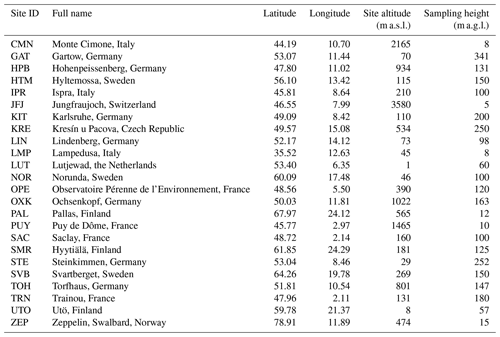Table 2The prior fluxes and δ13C value used for each source where the total and mean δ13C values are given for the European domain.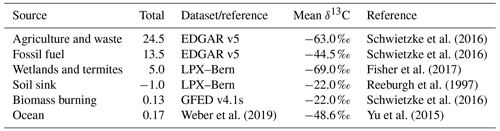The δ13C value is the ratio of 13C to 12C in a sample relative to a reference value measured in per mil (‰).

$\begin{array}{}\text{(17)}& {\mathit{\delta }}^{\mathrm{13}}\mathrm{C}=\left(\frac{{R}_{\mathrm{sam}}}{{R}_{\mathrm{ref}}}-\mathrm{1}\right)×\mathrm{1000}\end{array}$

The δ13C value in the atmosphere varies as a result of variations in the δ13C value of the sources, the oxidation of CH4 in the atmosphere and in soils, and atmospheric transport. Sources of CH4 can be grouped according to their characteristic δ13C value, with microbial sources being the most depleted in 13C, while thermogenic sources such as from oil, gas, and coal are less depleted, and pyrogenic sources, such as biomass burning, are the least depleted (Fisher et al., 2011; Dlugokencky et al., 2011; Brownlow et al., 2017) (Table 2). In this example, CH4 sources were grouped into anthropogenic microbial sources, namely agriculture and waste (agw); fossil sources, namely fossil fuel and geological emissions (fos); biomass burning sources (bbg); and natural microbial sources, principally wetlands (wet) and the ocean source (oce). The change in CH4 mixing ratios from all sources can thus be written as

$\begin{array}{}\text{(18)}& \mathrm{\Delta }c=\mathbf{H}{\mathbit{x}}_{\mathrm{agw}}+\mathbf{H}{\mathbit{x}}_{\mathrm{wet}}+\mathbf{H}{\mathbit{x}}_{\mathrm{fos}}+\mathbf{H}{\mathbit{x}}_{\mathrm{bbg}}+\mathbf{H}{\mathbit{x}}_{\mathrm{oce}},\end{array}$

where Δc is the change in CH4 mixing ratios, x is the vector of fluxes, and H is the transport operator. Analogously, the change in δ13C can be defined as

$\begin{array}{}\text{(19)}& \begin{array}{rl}\mathrm{\Delta }{\mathit{\delta }}^{\mathrm{13}}c& =\mathbf{H}{\mathit{\delta }}_{\mathrm{agw}}{\mathbit{x}}_{\mathrm{agw}}+\mathbf{H}{\mathit{\delta }}_{\mathrm{wet}}{\mathbit{x}}_{\mathrm{wet}}+\mathbf{H}{\mathit{\delta }}_{\mathrm{fos}}{\mathbit{x}}_{\mathrm{fos}}\\ & +\mathbf{H}{\mathit{\delta }}_{\mathrm{bbg}}{\mathbit{x}}_{\mathrm{bbg}}+\mathbf{H}{\mathit{\delta }}_{\mathrm{oce}}{\mathbit{x}}_{\mathrm{oce}},\end{array}\end{array}$

where δx is the isotopic signature for each source type. Therefore, the transport operator for an observation of the change in δ13C is just the transport operator H but scaled by δx for each source.

For this example, SRRs were calculated for all 24 sites in the ICOS network using the Lagrangian particle dispersion model, FLEXPART (Pisso et al., 2019), driven with ERA-Interim reanalysis wind fields. Retro-plumes were calculated for 10 d backwards in time from each site at an hourly frequency. The SRRs were saved at 0.5× 0.5 resolution over the European domain of 12 W to 32 E and 35 to 72 N and averaged over all observations within a month to give a monthly mean SRR for each site.

The uncertainty in the δ13C measurements was set to the same value for each site, that is, at 0.07 ‰ based on experimental values (Menoud et al., 2020). Similarly, the uncertainty in CH4 mixing ratio measurements was also set to the same value at all sites, at 5 ppb (WMO, 2009). The prior uncertainty σ for each grid cell was calculated as 0.5 times the prior flux, with a lower threshold equal to the first percentile value of all grid cells with non-zero flux for the smallest flux source. The spatial correlation between grid cells was calculated based on exponential decay over distance with a correlation scale length of 250 km over land. The prior error covariance matrix was then calculated as

$\begin{array}{}\text{(20)}& \mathbf{B}=\mathbf{\Sigma }\mathbf{C}\mathbf{\Sigma },\end{array}$

where C is the spatial correlation matrix and Σ is a diagonal matrix with the diagonal terms equal to the prior uncertainties for each grid cell.

For this example, the optimal network was found for three different scenarios: (1) monitoring all sources in EU27 countries plus the UK, Norway, and Switzerland (EU27 + 3); (2) monitoring only anthropogenic sources in EU27 + 3; and (3) as in scenario 1 but ignoring the existing information provided by CH4 mixing ratios at all sites.

For these scenarios the influence of the fluxes that are not the target of the network needs to be projected into the observation space and included in the R matrix. For example, in scenario 1 this is the influence of fluxes outside EU27 + 3, and in scenario 2 it is the influence of all non-anthropogenic sources plus the influence of fluxes outside EU27 + 3. This is calculated as

$\begin{array}{}\text{(21)}& \mathbf{R}={\mathbf{HB}}_{\mathrm{other}}{\mathbf{H}}^{\mathrm{T}}+{\mathbf{R}}_{\mathrm{meas}},\end{array}$

where Rmeas is simply the prior measurement uncertainty and Bother is the prior error covariance matrix for the other (i.e. non-target) fluxes.

For all scenarios the choice of the first four optimal sites was the same, that is, IPR, SAC, KIT, and LIN, while the last site chosen was KRE in scenarios 1 and 2 (Fig. 1) and LUT in scenario 3. All chosen sites are strongly sensitive to anthropogenic emissions, and the choice to optimize all sources or only anthropogenic sources made no difference in this example, likely because the natural sources (predominantly wetlands) are a relatively small contribution to the total CH4 source in Europe (only 12 %). On the other hand, ignoring existing information provided by CH4 mixing ratios led to LUT being chosen over KRE, likely because LUT provides a stronger constraint on the region with the largest emissions and diverse sources, i.e. Benelux (Fig. 2), which is more important in the absence of CH4 mixing ratio data.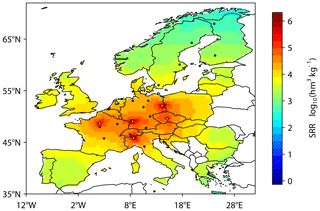Figure 1Map of the total mean SRRs for optimal sites for scenarios 1 and 2. Since only the EU27 + 3 emissions are constrained, the SRRs are also only shown for EU27 + 3. The locations of the optimal sites are indicated by the white points (i.e. IPR, SAC, KIT, LIN, and KRE), and the locations of the unselected sites are indicated by the black points.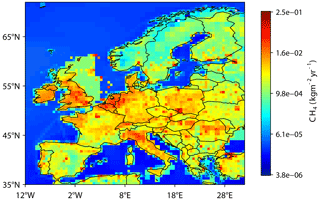Figure 2Map of annual mean CH4 emissions (units of kg m−2 yr−1) plotted with a logarithmic (base of 2) colour scale.

## 3.2 Network of 14CO2 measurements for fossil fuel emissions

This example concerns the establishment of a network for measurements of radiocarbon dioxide, 14CO2, which can be used as a tracer for fossil fuel CO2 emissions; since fossil fuel contains no 14C, its combustion depletes the atmospheric background value of 14CO2 (Turnbull et al., 2009). Similar to the previous example, the ICOS network is used, which also has CO2 measurements at 24 sites in Europe. The hypothetical problem can be formulated as follows: if there is a budget to equip 10 sites in the ICOS network with weekly flask samples for 14CO2 analysis, which sites should be chosen to gain the most knowledge of fossil fuel emissions? In this case, only weekly measurement frequency is examined as 14CO2 measurements cannot be made continuously, and the measurement method, either via counting radioactive decay or by accelerator mass spectrometry, is costly and time-consuming. The optimization problem needs to consider the information already brought by the CO2 measurements at all sites (in this example hourly measurements) and, in addition, the influence on the atmospheric signal from other sources, which may change the sensitivity of a site to fossil fuel emissions.

Measurements of 14CO2 are reported as the ratio of 14CO2 to CO2 relative to a reference ratio and given in units of per mil (‰).

$\begin{array}{}\text{(22)}& {\mathrm{\Delta }}^{\mathrm{14}}\mathrm{C}=\left(\frac{{R}_{\mathrm{sam}}}{{R}_{\mathrm{ref}}}-\mathrm{1}\right)×\mathrm{1000}\end{array}$

Since fossil fuels contain no 14C, its isotopic ratio is 1000 ‰. Other than fossil fuels, atmospheric values of Δ14CO2 are determined by the natural production of 14CO2 in the stratosphere, nuclear power and spent fuel-processing plants, ocean and land biosphere fluxes, and atmospheric transport. Ocean fluxes affect 14CO2, since the ocean is not in isotopic equilibrium with the atmosphere owing to higher values of atmospheric 14CO2 in the past due to nuclear bomb testing and similarly for plant respiration fluxes of CO2 (Bozhinova et al., 2014).

The change in CO2 mixing ratios can be described as follows:

$\begin{array}{}\text{(23)}& \mathrm{\Delta }c=\mathbf{H}{\mathbit{x}}_{\mathrm{fos}}+\mathbf{H}{\mathbit{x}}_{\mathrm{pho}}+\mathbf{H}{\mathbit{x}}_{\mathrm{res}}+\mathbf{H}{\mathbit{x}}_{\mathrm{oce}},\end{array}$

where xfos is the fossil fuel emission, xpho the land biosphere photosynthesis flux, xres the land biosphere respiration flux, and xoce the net ocean flux. A similar expression for the change in Δ14CO2 can be derived following Bozhinova et al. (2014) as

$\begin{array}{}\text{(24)}& \begin{array}{rl}{\mathrm{\Delta }}^{\mathrm{14}}c& =\mathbf{H}{\mathrm{\Delta }}_{\mathrm{fos}}{\mathbit{x}}_{\mathrm{fos}}+\mathbf{H}{\mathrm{\Delta }}_{\mathrm{pho}}{\mathbit{x}}_{\mathrm{pho}}+\mathbf{H}{\mathrm{\Delta }}_{\mathrm{res}}{\mathbit{x}}_{\mathrm{res}}\\ & +\mathbf{H}{\mathrm{\Delta }}_{\mathrm{oce}}{\mathbit{x}}_{\mathrm{oce}}+\mathbf{H}{\mathrm{\Delta }}_{\mathrm{nuc}}{\mathbit{x}}_{\mathrm{nuc}},\end{array}\end{array}$

where Δ14c is the change in Δ14CO2, the term Δx is the isotopic signature of the corresponding source, and HΔnucxnuc is the production of 14CO2 from nuclear facilities. There is a term missing from Eqs. (23) and (24), namely the stratospheric production of CO2 and 14CO2. This term is ignored as the direct stratospheric contribution is negligible for the time and space domain considered by the Lagrangian model, since the observations are close to the surface. Equation (24) can be further simplified by removing the term HΔphoxpho, since photosynthesis, although it affects the 14CO2 mixing ratio, does not affect Δ14CO2 (Turnbull et al., 2009). Furthermore, the ocean and respiration fluxes can be split into a background term and a disequilibrium term, Δbgocedis and Δbgresdis, respectively. As for photosynthesis, the background terms for ocean and respiration fluxes do not change Δ14CO2, but only the disequilibrium terms change Δ14CO2. For the domain in consideration, these terms are much smaller than that of fossil fuels and are ignored as in Bozhinova et al. (2014). With these simplifications, Eq. (24) becomes the following.

$\begin{array}{}\text{(25)}& {\mathrm{\Delta }}^{\mathrm{14}}c=\mathbf{H}{\mathrm{\Delta }}_{\mathrm{fos}}{\mathbit{x}}_{\mathrm{fos}}+\mathbf{H}{\mathrm{\Delta }}_{\mathrm{nuc}}{\mathbit{x}}_{\mathrm{nuc}}\end{array}$

Since xnuc is pure 14CO2, Δnuc would be infinite; therefore, the approach of Bozhinova et al. (2014) is used and Δnuc is approximated as the ratio of the activity of the sample and the referenced standard, giving ${\mathrm{\Delta }}_{\mathrm{nuc}}\approx \mathrm{0.7}×{\mathrm{10}}^{\mathrm{15}}$ ‰.

Because, in this example, only the fossil fuel emissions are the unknown variables and the target of the network, the matrix B corresponds only to the uncertainty in the fossil fuel emissions and is resolved monthly. The other terms influencing CO2 and Δ14CO2 are projected into the observation space and included in the R matrix using Eq. (21). For the Δ14CO2 observations, Bother is only the nuclear source, and for CO2 observations, Bother includes photosynthesis and respiration, the sum of which is net ecosystem exchange (NEE) and the ocean flux, for which the effect on the observed CO2 signal is very small and is thus ignored here. For both NEE and nuclear emissions, an uncertainty of 0.5 times the value in each grid cell was used to calculate Bother with a spatial correlation length of 250 km. Since NEE fluxes have large diurnal and seasonal cycles which co-vary with atmospheric transport, for the CO2 observations, R was calculated using H and B, resolved for day, night, and monthly. Note, only one uncertainty value was calculated for each site, which represents the annual mean uncertainty for a daytime observation. Each site has a different uncertainty for CO2 mixing ratios and Δ14CO2 depending on the influence of NEE fluxes and nuclear emissions, respectively. This can be simply interpreted in terms of a signal-to-noise ratio. For example, for CO2 mixing ratios where there is a large influence of NEE, the time series becomes noisier, similarly for the influence of nuclear emissions on Δ14CO2 observations. The measurement uncertainty, Rmeas, was set to the same value for each site, that is, at 2 ‰ for Δ14CO2 (Turnbull et al., 2007) and 0.05 ppm for CO2 mixing ratio measurements (WMO, 2018).

For this example, SRRs were calculated for all 24 sites in the ICOS network using FLEXPART with retro-plumes calculated for 5 d backwards in time from each site at an hourly frequency. The SRRs were saved at 0.5× 0.5 and 3-hourly resolutions over the European domain of 15 W to 35 E and 30 to 75 N and were averaged to give mean day and night SRRs for each month for each site. Estimates of NEE fluxes were used from the Simple Biosphere Model – Carnegie Ames Stanford Approach (SiBCASA) and were resolved 3-hourly (Schaefer et al., 2008), estimates of nuclear emissions were used from the CO2 human emissions (CHE) project (Potier et al., 2022) and were an annual climatology, and estimates of fossil fuel emissions were from GridFED at monthly resolution (Jones et al., 2020a).

Figure 3 shows the uncertainty in the observation space at each site due to the influence of uncertainties in NEE and nuclear emissions on CO2 mixing ratios and Δ14CO2 values, respectively. For CO2, sites in western Europe have the largest uncertainties, while sites in northern Scandinavia and southern Europe have smaller uncertainties following the pattern of NEE amplitude. For Δ14CO2, most sites have only small uncertainties owing to nuclear emissions, but two notable exceptions are NOR and KIT, and both are close to large nuclear sources.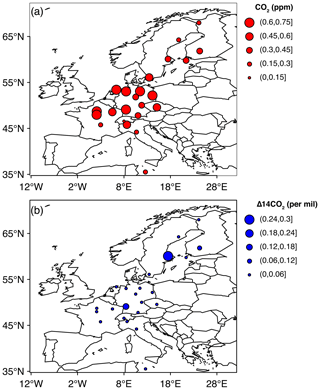Figure 3Maps showing the uncertainty at each site from the projection of flux uncertainty into the observation space, (a) CO2 mixing ratios and (b) Δ14CO2.

The optimal sites in the order selected are SAC, KIT, LUT, KRE, STE, LIN, GAT, IPR, TRN, and TOH (Fig. 4). Two of the sites, SAC and TRN, are relatively close to one another (approximately 95 km apart); however, they have somewhat different footprints with SAC sampling more of the Paris region and TRN sampling more of the south and east. If the prior error covariance matrix, B, and the transport operator, H, are not resolved monthly but only annually, the optimal sites differ by only one site, namely HPB instead of TRN. If the existing information provided by CO2 mixing ratios is ignored (i.e. the network is designed only considering information from Δ14CO2), then the choice of optimal sites differs slightly, and TRN and TOH are no longer selected but OPE and LMP. The choice of LMP may seem unexpected at first, but it is close to an emission hotspot in Tunis, Tunisia (Fig. 5). The reason this site is not selected when the information from CO2 mixing ratios is included is presumably because the CO2 mixing ratio already provides a reasonable constraint on the fossil fuel emissions with the NEE signal being relatively small.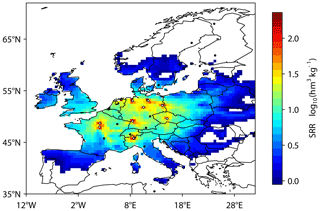Figure 4Map of the total mean SRR for optimal sites for monitoring fossil fuel CO2 emissions with monthly resolution and including existing information from CO2 mixing ratios. The locations of the optimal sites are indicated by the white points (i.e. SAC, KIT, LUT, KRE, STE, LIN, GAT, IPR, TRN, and TOH), and the locations of the unselected sites are indicated by the black points.Figure 5Map of annual mean fossil fuel CO2 emissions (units of kg m−2 yr−1) plotted with a logarithmic (base of 2) colour scale.

4 Discussion

An obvious question is how does the criterion of information content compare to criteria based on the posterior uncertainty? The information content describes the change in probability space from before an observation is made (prior probability) compared to after an observation is made (posterior probability) and is thus more closely linked to the observations themselves than to the exact definition of the posterior uncertainty metric. The performance of the two metrics, i.e. information content versus the sum of the posterior error covariance matrix, was examined using the CH4 example in scenario 1 (described in Sect. 3.1). For this example, a second network was selected using the criterion of the sum of the posterior error covariance matrix and consisted of the sites HPB, HTM, KRE, PUY, and TRN (only KRE was also selected using the information criterion). Two inversions were performed using pseudo-observations generated by applying the transport operator, H (with rows corresponding to daily afternoon means for each site and columns corresponding to the six source types resolved annually), to the annual mean fluxes for each source type, x, and adding random noise according to the error characteristics of R.

$\begin{array}{}\text{(26)}& {\mathbit{y}}_{\mathrm{obs}}=\mathbf{H}\mathbit{x}+{\mathbf{R}}^{\frac{\mathrm{1}}{\mathrm{2}}}r\phantom{\rule{1em}{0ex}}\mathrm{where}\phantom{\rule{0.25em}{0ex}}r\sim N\left(\mathrm{0},\mathrm{1}\right)\end{array}$

In these inversions, the prior was generated by randomly perturbing the fluxes according to the error characteristics of B.

$\begin{array}{}\text{(27)}& {\mathbit{x}}_{\mathrm{b}}=\mathbit{x}+{\mathbf{B}}^{\frac{\mathrm{1}}{\mathrm{2}}}r\phantom{\rule{1em}{0ex}}\mathrm{where}\phantom{\rule{0.25em}{0ex}}r\sim N\left(\mathrm{0},\mathrm{1}\right)\end{array}$

Both inversions used the same prior fluxes and uncertainties and differed only in the set of sites used. The performance of the inversions was tested using the so-called gain metric, G, based on the ratio of the distance of the posterior from the true fluxes to the distance of the prior from the true fluxes:

$\begin{array}{}\text{(28)}& G=\mathrm{1}-\sqrt{\frac{{\left(\mathbit{x}-{\mathbit{x}}_{\mathrm{a}}\right)}^{\mathrm{2}}}{{\left(\mathbit{x}-{\mathbit{x}}_{\mathrm{b}}\right)}^{\mathrm{2}}}},\end{array}$

where xa is the posterior flux vector. The larger the value of G, the closer the posterior is to the true flux. Using the optimal sites according to the information content G=0.6996, while using the optimal sites according to the posterior error covariance G=0.6988. (A comparison of the prior and posterior compared to the true fluxes is shown in Fig. 6.) Thus, the information content performs at least as well as the posterior error covariance metric for determining a network.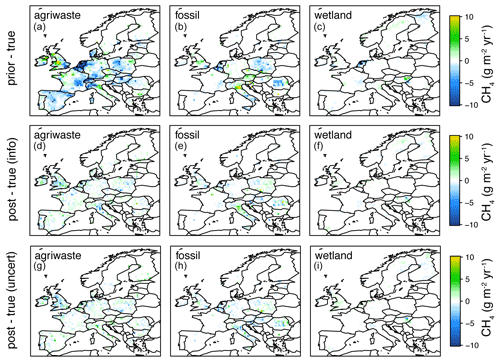Figure 6Comparison of the true-prior flux differences (units g m−2 yr−1(a–c) versus the true-posterior flux differences for the inversion using sites chosen with the information content criterion (d–f) and the inversion using sites chosen with the posterior uncertainty criterion (g–i). Note: only the fluxes for the sources agriculture and waste, fossil, and biomass burning sources are shown as the other three sources are very minor.

Another question that arises is how does this method compare to methods based on the analysis of the variability in the time series at the different sites? To answer this question, a clustering method was applied to the example of designing a network for fossil fuel CO2 emissions. For this, a time series of Δ14CO2 was generated for each of the 24 sites using Eq. (25) (see the Supplement for plots of the time series). The values were generated hourly, but, since generally only daytime values are used in inverse modelling, data were selected for the time interval 12:00 to 15:00 LT​​​​​​​. A dissimilarity matrix was calculated for the 24 time series (using the R function proxy::dist with the dynamic time warp (DTW) method; Giorgino, 2009). The divisive hierarchical clustering method (R function cluster::diana) was applied to the dissimilarity matrix, stopping at 10 clusters. The first cluster contained 13 sites, that is, those with little signal (e.g. JFJ, CMN, and ZEP). Two clusters contained two sites, namely IPR and KRE, as well as OPE and TRN, while the remaining clusters contained only one site. Based on the principle of choosing sites that display different signals, one would choose the sites which are in a cluster of one. This would lead to the choice of GAT, KIT, LIN, LUT, SAC, STE, and TOH. These seven sites are also chosen by the method based on information content. However, the question is how to choose the remaining three sites from clusters with more than one site. For this there is no single answer. Moreover, the sites that are the most dissimilar are not necessarily those that will provide the most information about the target fluxes of the network, since the reasons for dissimilarity are various, e.g. having little signal, being sensitive to sources that are not the target of the network, or owing to distinct atmospheric circulation patterns. Sites with high degrees of similarity may both offer a strong constraint and both be valuable to a network (in this example IPR and KRE were in the same cluster, but both sites are chosen in the method based on information content).

In the examples presented, the atmospheric transport matrix, H, and the matrix, B, were resolved at 0.5× 0.5 (and considered only land grid cells) and monthly. The size of the matrix B (and the matrix ${\mathbf{H}}^{\mathrm{T}}{\mathbf{R}}^{-\mathrm{1}}\mathbf{H}+{\mathbf{B}}^{-\mathrm{1}}$) for the example of a network for fossil fuel CO2 emissions was  11  Gb. However, in the case of finer spatial resolution or a larger domain, which means the size of the matrices exceeds the available memory, it is still possible to use this method as long as B and ${\mathbf{H}}^{\mathrm{T}}{\mathbf{R}}^{-\mathrm{1}}\mathbf{H}+{\mathbf{B}}^{-\mathrm{1}}$ defined for one time step do not exceed the memory. In this case, the problem can be solved by summing the information content calculated separately for each time step. Disaggregating the problem in this way does not lead to the same value of information content as when all time steps are considered together; however, the choice of sites is nearly the same. For the example of a network for fossil fuel CO2 emissions, the two methods (i.e. disaggregating versus not disaggregating) differed by only one site. For the example of a fossil fuel network, the total computation time was  3 h using multi-threaded parallelization on eight cores.

In addition to the memory requirements, there is the question of the computational cost determined by the complexity of the algorithm, in particular compared to the more established method using a metric based on the posterior error covariance. Such analysis can be performed putting aside the practical considerations related to particular software and/or hardware. It has been established that the algorithmic complexity, and hence the computational cost, of the calculation of the determinant is the same as that of matrix multiplication (Strassen, 1969; Aho et al., 1974). Ignoring the particularities of the algorithm used and its hardware implementation, the analysis can be simplified by counting the number of matrix multiplications: O(mnk) for two generic rectangular matrices, Cholesky decompositions ($O\left({n}^{\mathrm{3}}\right)/\mathrm{3}$), matrix inversions, and determinant calculations (both obtained e.g. from the Cholesky decomposition). Both the error covariance metric and the information metric require the inversion of the matrix B. The covariance metric requires the Hessian matrix $\mathbf{G}={\mathbf{H}}^{\mathrm{T}}{\mathbf{R}}^{-\mathrm{1}}\mathbf{H}+{\mathbf{B}}^{-\mathrm{1}}$that takes one inversion of B ($\sim O\left({n}^{\mathrm{3}}\right)/\mathrm{3}$), one inversion of R ($\sim O\left({k}^{\mathrm{3}}\right)/\mathrm{3}$), and the product of three matrices ($O\left(n{k}^{\mathrm{2}}+{n}^{\mathrm{2}}k\right)\sim O\left({n}^{\mathrm{2}}\right)$ if kn). This yields G in $O\left({n}^{\mathrm{3}}\right)/\mathrm{3}+O\left({k}^{\mathrm{3}}\right)/\mathrm{3}+O\left(n{k}^{\mathrm{2}}+{n}^{\mathrm{2}}k\right)$ operations. The inverse of G yields the posterior covariance in $O\left({k}^{\mathrm{3}}\right)/\mathrm{3}$ operations via the Cholesky decomposition. Subsequent steps are of lower computational order. Even if some simplifications are possible, its complexity is bounded below by $\mathrm{2}O\left({n}^{\mathrm{3}}\right)/\mathrm{3}$. Therefore, the information metric is not computationally more expensive than the covariance metric. The algorithm presented here is faster than the naive computation of the information content from its formal definition.

5 Conclusions

A method for designing atmospheric observation networks is presented based on information theory. This method can be applied to any type of atmospheric data: mixing ratios, aerosols, isotopic ratios, and total column measurements. In addition, the method allows the network to be designed with or without considering existing information, which may also be of a different type, e.g. mixing ratios of a different species or isotopic ratios. Since the method does not require inverting any large matrices (e.g. for the calculation of posterior uncertainties), and the calculation of B−1 only needs to be performed once, it is very efficient and can also be used on large problems. The only constraint is that the matrices B and HTR−1H + B−1 defined for one time step do not exceed the available memory. The algorithm allows the exact problem to be defined, that is, to target specific emission sources or regions. Two examples are presented: the first is to select sites from an existing network of CH4 mixing ratios for additional measurements of δ13C to constrain emissions in EU countries (plus Norway, Switzerland, and the UK), and the second is to select sites from an existing network of CO2 mixing ratios for additional measurements of Δ14CO2 to monitor fossil fuel CO2 emissions. These examples demonstrated that the optimal network differs depending on its exact purpose, e.g. is the network targeting emissions over the whole domain or for a specific region? And should existing information be considered or not? Thus it is important that the method of network design is able to account for these considerations.

Code availability

The R code for the network design algorithm presented in this paper is available from Zenodo: https://doi.org/10.5281/zenodo.7070622 (Thompson and Pisso, 2022).

Data availability

The CH4 emissions data for anthropogenic sources are available from the EDGAR website (http://data.europa.eu/89h/488dc3de-f072-4810-ab83-47185158ce2a, last access: 12 January 2023; Crippa et al., 2019). The biomass burning sources are available from the GFED website (https://www.geo.vu.nl/~gwerf/GFED/GFED4/, last access: 12 January 2023; van der Werf et al., 2017). The wetland sources and soil sinks from the LPX-Bern model are available on request to Jurek Müller (jurek.mueller@unibe.ch), and the ocean sources are available from the website (https://doi.org/10.6084/m9.figshare.9034451.v1, Weber, 2019). The CO2 emissions data for anthropogenic sources are available from Zenodo (https://doi.org/10.5281/zenodo.3958283, Jones et al., 2020b). The NEE fluxes from the SiBCASA model are available on request to Ingrid Van der Laan (ingrid.vanderlaan@wur.nl), and the nuclear emissions estimates of 14CO2 are available on request to Elise Potier (elise.potier@lsce.ipsl.fr).

Supplement

Author contributions

RLT developed the algorithm, wrote the code, and carried out the examples. IP contributed to the modelling of Δ14CO2 and to the algorithm, performed the algorithm complexity analysis, and provided feedback on the paper.

Competing interests

The contact author has declared that neither of the authors has any competing interests.

Disclaimer

Publisher's note: Copernicus Publications remains neutral with regard to jurisdictional claims in published maps and institutional affiliations.

Acknowledgements

This work was supported by the European Commission, Horizon 2020 Framework Programme (VERIFY, grant no. 776810). We would like to acknowledge Jurek​​​​​​​ Müller for preparing the LPX–Bern simulations of wetland CH4 fluxes, as well as Elise Potier and Yilong Wang for providing the estimates of nuclear emissions of 14CO2.

Financial support

This research has been supported by the European Commission, Horizon 2020 Framework Programme (VERIFY, grant no. 776810).

Review statement

This paper was edited by Thomas Röckmann and reviewed by Peter Rayner and one anonymous referee.

References

Aho, A. V., Hopcroft, J. E., and Ullman, J. D.: The Design and Analysis of Computer Algorithms, Addison-Wesley, ISBN 978-0-201-00029-0, 1974.

Bozhinova, D., van der Molen, M. K., van der Velde, I. R., Krol, M. C., van der Laan, S., Meijer, H. A. J., and Peters, W.: Simulating the integrated summertime Δ14CO2 signature from anthropogenic emissions over Western Europe, Atmos. Chem. Phys., 14, 7273–7290, https://doi.org/10.5194/acp-14-7273-2014, 2014.

Brownlow, R., Lowry, D., Fisher, R. E., France, J. L., Lanoisellé, M., White, B., Wooster, M. J., Zhang, T., and Nisbet, E. G.: Isotopic ratios of tropical methane emissions by atmospheric measurement, Global Biogeochem. Cy., 31, 2017GB005689, https://doi.org/10.1002/2017gb005689, 2017.

Crippa, M., Guizzardi, D., Muntean, M., Schaaf, E., Lo Vullo, E., Solazzo, E., Monforti-Ferrario, F., Olivier, J., and Vignati, E.: EDGAR v5.0 Greenhouse Gas Emissions, European Commission, Joint Research Centre (JRC) [data set], http://data.europa.eu/89h/488dc3de-f072-4810-ab83-47185158ce2a (last access: 12 January 2023), 2019.

Dlugokencky, E. J., Nisbet, E. G., Fisher, R., and Lowry, D.: Global atmospheric methane: budget, changes and dangers, Phil. Trans. Roy. Soc., 369, 2058–2072, https://doi.org/10.1098/rsta.2010.0341, 2011.

Enting, I. G.: Inverse Problems in Atmospheric Constituent Transport, Cambridge University Press, Cambridge, ISBN 978-0-511-53574-1 , 2002.

Fisher, R. E., Sriskantharajah, S., Lowry, D., Lanoisellé, M., Fowler, C. M. R., James, R. H., Hermansen, O., Myhre, C. L., Stohl, A., Greinert, J., Nisbet-Jones, P. B. R., Mienert, J., and Nisbet, E. G.: Arctic methane sources: Isotopic evidence for atmospheric inputs, Geophys. Res. Lett., 38, L21803, https://doi.org/10.1029/2011gl049319, 2011.

Fisher, R. E., France, J. L., Lowry, D., Lanoisellé, M., Brownlow, R., Pyle, J. A., Cain, M., Warwick, N., Skiba, U. M., Drewer, J., Dinsmore, K. J., Leeson, S. R., Bauguitte, S. J. B., Wellpott, A., O'Shea, S. J., Allen, G., Gallagher, M. W., Pitt, J., Percival, C. J., Bower, K., George, C., Hayman, G. D., Aalto, T., Lohila, A., Aurela, M., Laurila, T., Crill, P. M., McCalley, C. K., and Nisbet, E. G.: Measurement of the 13C isotopic signature of methane emissions from Northern European wetlands, Global Biogeochem. Cy., 31, 2016GB005504, https://doi.org/10.1002/2016gb005504, 2017.

Giorgino, T.: Computing and Visualizing Dynamic Time Warping Alignments in R: The dtw Package, J. Stat. Softw., 31, 1–24, https://doi.org/10.18637/jss.v031.i07, 2009.

Gloor, M., Fan, S., Pacala, S., and Sarmiento, J.: Optimal sampling of the atmosphere for purpose of inverse modeling: A model study, Global Biogeochem. Cy., 14, 407–428, 2000.

Hardt, M. and Scherbaum, F.: The design of optimum networks for aftershock recordings, Geophys. J. Int., 117, 716–726, https://doi.org/10.1111/j.1365-246X.1994.tb02464.x, 1994.

Jones, M. W., Andrew, R. M., Peters, G. P., Janssens-Maenhout, G., De-Gol, A. J., Ciais, P., Patra, P. K., Chevallier, F., De-Gol, A. J., Ciais, P., Patra, P. K., and Le Quéré, C.​​​​​​​: Gridded fossil CO2 emissions and related O2 combustion consistent with national inventories 1959–2018, Scientific Data, 8, 1–23, https://doi.org/10.1038/s41597-020-00779-6, 2020a.

Jones, M. W., Andrew, R. M., Peters, G. P., Janssens-Maenhout, G., De-Gol, A. J., Ciais, P., Patra, P. K., Chevallier, F., and Le Quéré, C.: Gridded fossil CO2 emissions and related O2 combustion consistent with national inventories 1959–2018 (GCP-GridFEDv2019.1), Zenodo [data set], https://doi.org/10.5281/zenodo.3958283, 2020b.

Lucas, D. D., Yver Kwok, C., Cameron-Smith, P., Graven, H., Bergmann, D., Guilderson, T. P., Weiss, R., and Keeling, R.: Designing optimal greenhouse gas observing networks that consider performance and cost, Geosci. Instrum. Method. Data Syst., 4, 121–137, https://doi.org/10.5194/gi-4-121-2015, 2015.

Menoud, M., van der Veen, C., Scheeren, B., Chen, H., Szénási, B., Morales, R. P., Pison, I., Bousquet, P., Brunner, D., and Röckmann, T.: Characterisation of methane sources in Lutjewad, The Netherlands, using quasi-continuous isotopic composition measurements, Tellus B, 72, 1–20, https://doi.org/10.1080/16000889.2020.1823733, 2020.

Nickless, A., Ziehn, T., Rayner, P. J., Scholes, R. J., and Engelbrecht, F.: Greenhouse gas network design using backward Lagrangian particle dispersion modelling – Part 2: Sensitivity analyses and South African test case, Atmos. Chem. Phys., 15, 2051–2069, https://doi.org/10.5194/acp-15-2051-2015, 2015.

Patra, P. K. and Maksyutov, S.: Incremental approach to the optimal network design for CO2 surface source inversion, Geophys. Res. Lett., 29, 97-1–97-4, https://doi.org/10.1029/2001gl013943, 2002.

Pisso, I., Sollum, E., Grythe, H., Kristiansen, N. I., Cassiani, M., Eckhardt, S., Arnold, D., Morton, D., Thompson, R. L., Groot Zwaaftink, C. D., Evangeliou, N., Sodemann, H., Haimberger, L., Henne, S., Brunner, D., Burkhart, J. F., Fouilloux, A., Brioude, J., Philipp, A., Seibert, P., and Stohl, A.: The Lagrangian particle dispersion model FLEXPART version 10.4, Geosci. Model Dev., 12, 4955–4997, https://doi.org/10.5194/gmd-12-4955-2019, 2019.

Potier, E., Broquet, G., Wang, Y., Santaren, D., Berchet, A., Pison, I., Marshall, J., Ciais, P., Bréon, F.-M., and Chevallier, F.: Complementing XCO2 imagery with ground-based CO2 and 14CO2 measurements to monitor CO2 emissions from fossil fuels on a regional to local scale, Atmos. Meas. Tech., 15, 5261–5288, https://doi.org/10.5194/amt-15-5261-2022, 2022.

Rayner, P. J., Enting, I. G., and Trudinger, C. M.: Optimizing the CO2 observing network for constraining sources and sinks, Tellus B, 48, 433–444, 1996.

Rayner, P. J., Enting, I. G., Francey, R. J., and Langenfelds, R.: Reconstructing the recent carbon cycle from atmospheric CO2, δ13C and O2/N2 observations, Tellus B, 51, 213–232, https://doi.org/10.3402/tellusb.v51i2.16273, 1999.​​​​​​​

Reeburgh, W. S., Hirsch, A. I., Sansone, F. J., Popp, B. N., and Rust, T. M.: Carbon kinetic isotope effect accompanying microbial oxidation of methane in boreal forest soils, Geochim. Cosmochim. Ac., 61, 4761–4767, 1997.

Risch, M. R., Kenski, D. M., and Gay, D. A.: A Great Lakes Atmospheric Mercury Monitoring network: Evaluation and design, Atmos. Environ., 85, 109–122, https://doi.org/10.1016/j.atmosenv.2013.11.050, 2014.

Rodgers, C. D.: Inverse methods for atmospheric sounding: theory and practice, World Scientific, https://doi.org/10.1142/3171, 2000.

Schaefer, K., Collatz, G. J., Tans, P., Denning, A. S., Baker, I., Berry, J., Prihodko, L., Suits, N., and Philpott, A.: Combined Simple Biosphere/Carnegie-Ames-Stanford Approach terrestrial carbon cycle model, J. Geophys. Res., 113, 187, G03034, https://doi.org/10.1029/2007jg000603, 2008.

Schwietzke, S., Sherwood, O. A., Bruhwiler, L. M. P., Miller, J. B., Etiope, G., Dlugokencky, E. J., Michel, S. E., Arling, V. A., Vaughn, B. H., White, J. W. C., and Tans, P. P.: Upward revision of global fossil fuel methane emissions based on isotope database, Nature, 538, 88–91, https://doi.org/10.1038/nature19797, 2016.

Seibert, P. and Frank, A.: Source-receptor matrix calculation with a Lagrangian particle dispersion model in backward mode, Atmos. Chem. Phys., 4, 51–63, https://doi.org/10.5194/acp-4-51-2004, 2004.

Shiga, Y. P., Michalak, A. M., Kawa, S. R., and Engelen, R. J.: In-situ CO2 monitoring network evaluation and design: A criterion based on atmospheric CO2 variability, J. Geophys. Res.-Atmos., 118, 2007–2018, https://doi.org/10.1002/jgrd.50168, 2013.

Strassen, V.: Gaussian Elimination is not Optimal, Numer. Math., 13, 354–356, https://doi.org/10.1007/BF02165411, 1969.

Tarantola, A.: Inverse problem theory and methods for model parameter estimation, Society for Industrial and Applied Mathematics, ISBN 978-0-89871-572-9, 2005.

Thompson, R. and Pisso, I.: A Flexible Algorithm for Network Design Based on Information Theory, Zenodo [code], https://doi.org/10.5281/zenodo.7070622, 2022.

Turnbull, J., Rayner, P., Miller, J., Naegler, T., Ciais, P., and Cozic, A.: On the use of 14CO2 as a tracer for fossil fuel CO2: Quantifying uncertainties using an atmospheric transport model, J. Geophys. Res.-Atmos., 114, D22302, https://doi.org/10.1029/2009jd012308, 2009.

Turnbull, J. C., Lehman, S. J., Miller, J. B., Sparks, R. J., Southon, J. R., and Tans, P. P.: A new high precision 14CO2 time series for North American continental air, J. Geophys. Res.-Atmos., 112, D11310, https://doi.org/10.1029/2006jd008184, 2007.

van der Werf, G. R., Randerson, J. T., Giglio, L., van Leeuwen, T. T., Chen, Y., Rogers, B. M., Mu, M., van Marle, M. J. E., Morton, D. C., Collatz, G. J., Yokelson, R. J., and Kasibhatla, P. S.: Global fire emissions estimates during 1997–2016, Earth Syst. Sci. Data, 9, 697–720, https://doi.org/10.5194/essd-9-697-2017, 2017 (https://www.geo.vu.nl/~gwerf/GFED/GFED4/, last access: 12 January 2023).

Weber, T.: ocean_ch4.nc, figshare [data set], https://doi.org/10.6084/m9.figshare.9034451.v1, 2019.

Weber, T., Wiseman, N. A., and Kock, A.: Global ocean methane emissions dominated by shallow coastal waters, Nat. Commun., 10, 1–10, https://doi.org/10.1038/s41467-019-12541-7, 2019.

WMO: Guidelines for the Measurement of Methane and Nitrous Oxide and their Quality Assurance, GAW Report No. 185, Geneva, Switzerland, https://library.wmo.int/index.php?lvl=notice_display&id=139#.Y8E2ay0w3Fw (last access: 12 January 2023), 2009.

WMO: 19th WMO/IAEA Meeting on Carbon Dioxide, Other Greenhouse Gases and Related Measurement Techniques (GGMT-2017), Geneva, Switzerland, https://library.wmo.int/index.php?lvl=notice_display&id=20698#.Y8E3AS0w3Fw (last access: 12 January 2023), 2018.

Yu, J., Xie, Z., Sun, L., Kang, H., He, P., and Xing, G.: δ13C-CH4 reveals CH4 variations over oceans from mid-latitudes to the Arctic, Sci. Rep., 5, 1–9, https://doi.org/10.1038/srep13760, 2015.